Anzeige

# I.S.C. Class XII Sample Papers 2016

26. Feb 2016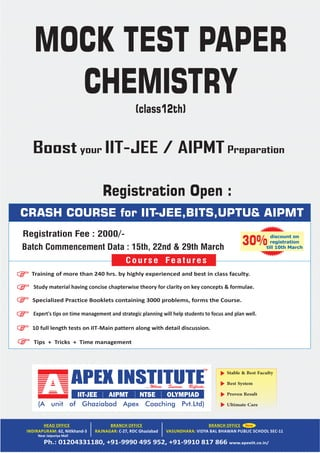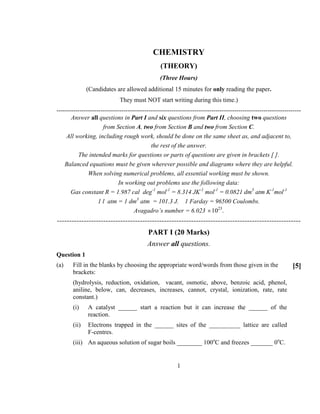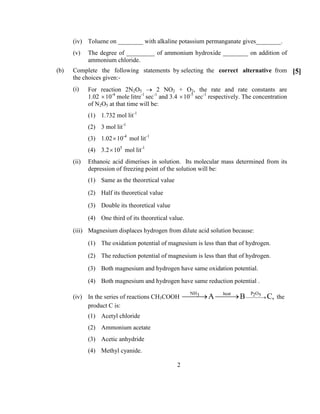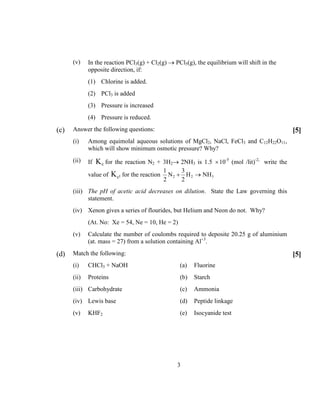Anzeige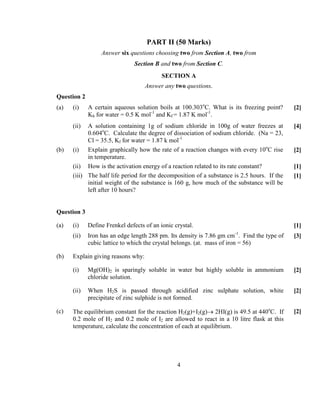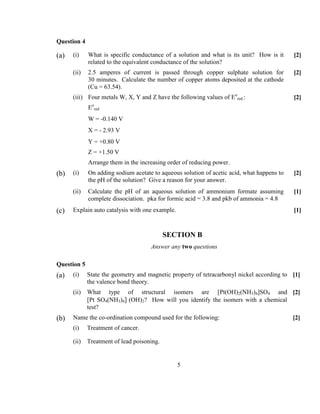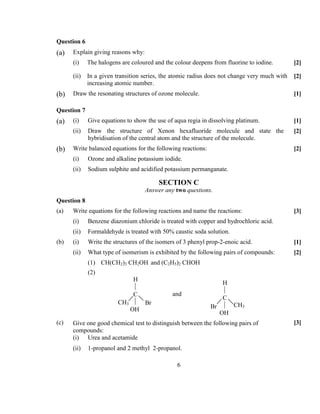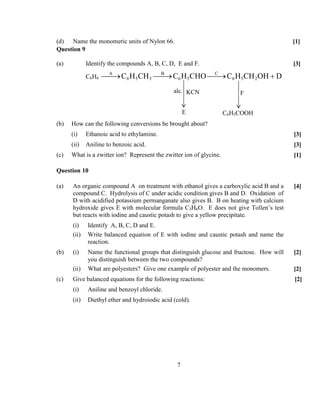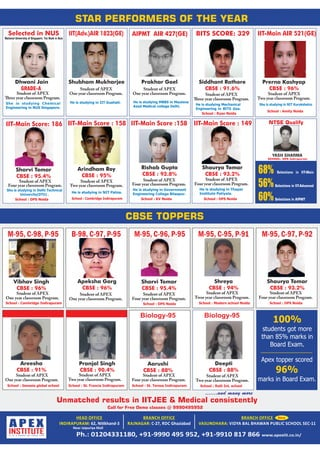Nächste SlideShare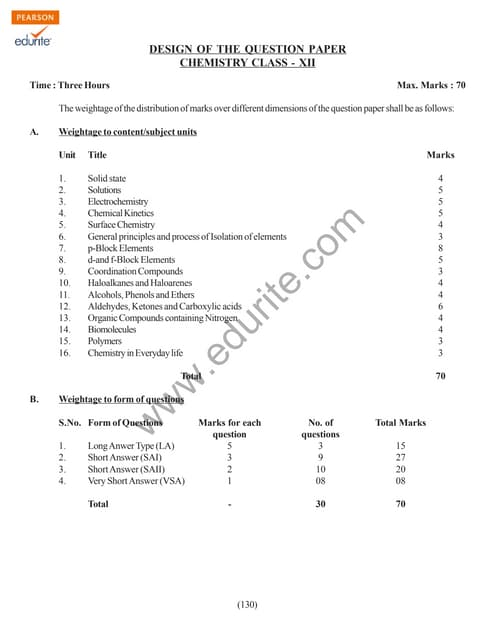Class 12 Cbse Chemistry Sample Paper 2010 Model 1
1 von 9
Anzeige

### I.S.C. Class XII Sample Papers 2016

1. Stable & Best Faculty Best System Proven Result Ultimate Care Near Jaipuriya Mall CRASH COURSE for IIT-JEE,BITS,UPTU& AIPMT Tips + Tricks + Time management Training of more than 240 hrs. by highly experienced and best in class faculty. Study material having concise chapterwise theory for clarity on key concepts & formulae. Specialized Practice Booklets containing 3000 problems, forms the Course. Expert's tips on time management and strategic planning will help students to focus and plan well. 10 full length tests on IIT‐Main pattern along with detail discussion. Course Features discount on registration till 10th March 30% MOCK TEST PAPER CHEMISTRY (class12th) Registration Fee : 2000/- Registration Open : Batch Commencement Data : 15th, 22nd & 29th March Boost your IIT-JEE / AIPMT Preparation
2. 1 CHEMISTRY (THEORY) (Three Hours) (Candidates are allowed additional 15 minutes for only reading the paper. They must NOT start writing during this time.) --------------------------------------------------------------------------------------------------------------------- Answer all questions in Part I and six questions from Part II, choosing two questions from Section A, two from Section B and two from Section C. All working, including rough work, should be done on the same sheet as, and adjacent to, the rest of the answer. The intended marks for questions or parts of questions are given in brackets [ ]. Balanced equations must be given wherever possible and diagrams where they are helpful. When solving numerical problems, all essential working must be shown. In working out problems use the following data: Gas constant R = 1.987 cal deg-1 mol-1 = 8.314 JK-1 mol-1 = 0.0821 dm3 atm K-1 mol-1 1 l atm = 1 dm3 atm = 101.3 J. 1 Farday = 96500 Coulombs. Avagadro’s number = 6.023 ×1023 . ---------------------------------------------------------------------------------------------------- PART I (20 Marks) Answer all questions. Question 1 (a) Fill in the blanks by choosing the appropriate word/words from those given in the brackets: (hydrolysis, reduction, oxidation, vacant, osmotic, above, benzoic acid, phenol, aniline, below, can, decreases, increases, cannot, crystal, ionization, rate, rate constant.)  (i) A catalyst ______ start a reaction but it can increase the ______ of the reaction. (ii) Electrons trapped in the ______ sites of the __________ lattice are called F-centres. (iii) An aqueous solution of sugar boils ________ 100o C and freezes _______ 0o C.
3. 2 (iv) Toluene on ________ with alkaline potassium permanganate gives________. (v) The degree of _________ of ammonium hydroxide ________ on addition of ammonium chloride. (b) Complete the following statements by selecting the correct alternative from the choices given:-  (i) For reaction 2N2O5 → 2 NO2 + O2, the rate and rate constants are 1.02 ×10-4 mole litre-1 sec-1 and 3.4 ×10-5 sec-1 respectively. The concentration of N2O5 at that time will be: (1) 1.732 mol lit-1 (2) 3 mol lit-1 (3) 1.02×10-4 mol lit-1 (4) 3.2×105 mol lit-1 (ii) Ethanoic acid dimerises in solution. Its molecular mass determined from its depression of freezing point of the solution will be: (1) Same as the theoretical value (2) Half its theoretical value (3) Double its theoretical value (4) One third of its theoretical value. (iii) Magnesium displaces hydrogen from dilute acid solution because: (1) The oxidation potential of magnesium is less than that of hydrogen. (2) The reduction potential of magnesium is less than that of hydrogen. (3) Both magnesium and hydrogen have same oxidation potential. (4) Both magnesium and hydrogen have same reduction potential . (iv) In the series of reactions CH3COOH NH P Oheat3 2 5 A B C,→→ → the product C is: (1) Acetyl chloride (2) Ammonium acetate (3) Acetic anhydride (4) Methyl cyanide.
4. 3 (v) In the reaction PCl3(g) + Cl2(g) → PCl5(g), the equilibrium will shift in the opposite direction, if: (1) Chlorine is added. (2) PCl3 is added (3) Pressure is increased (4) Pressure is reduced. (c) Answer the following questions:  (i) Among equimolal aqueous solutions of MgCl2, NaCl, FeCl3 and C12H22O11, which will show minimum osmotic pressure? Why? (ii) If cK for the reaction N2 + 3H2→ 2NH3 is 1.5 ×10-5 (mol /lit)-2, write the value of 1cK for the reaction 2 2 3 1 3 N H NH 2 2 + → (iii) The pH of acetic acid decreases on dilution. State the Law governing this statement. (iv) Xenon gives a series of flourides, but Helium and Neon do not. Why? (At. No: Xe = 54, Ne = 10, He = 2) (v) Calculate the number of coulombs required to deposite 20.25 g of aluminium (at. mass = 27) from a solution containing Al+3 . (d) Match the following:  (i) CHCl3 + NaOH (a) Fluorine (ii) Proteins (b) Starch (iii) Carbohydrate (c) Ammonia (iv) Lewis base (d) Peptide linkage (v) KHF2 (e) Isocyanide test
5. 4 PART II (50 Marks) Answer six questions choosing two from Section A, two from Section B and two from Section C. SECTIO A Answer any two questions. Question 2 (a) (i) A certain aqueous solution boils at 100.303o C. What is its freezing point? Kb for water = 0.5 K mol-1 and Kf = 1.87 K mol-1 .  (ii) A solution containing 1g of sodium chloride in 100g of water freezes at 0.604o C. Calculate the degree of dissociation of sodium chloride. (Na = 23, Cl = 35.5, Kf for water = 1.87 k mol-1  (b) (i) Explain graphically how the rate of a reaction changes with every 10o C rise in temperature.  (ii) How is the activation energy of a reaction related to its rate constant?  (iii) The half life period for the decomposition of a substance is 2.5 hours. If the initial weight of the substance is 160 g, how much of the substance will be left after 10 hours?  Question 3 (a) (i) Define Frenkel defects of an ionic crystal.  (ii) Iron has an edge length 288 pm. Its density is 7.86 gm cm-1 . Find the type of cubic lattice to which the crystal belongs. (at. mass of iron = 56)  (b) Explain giving reasons why: (i) Mg(OH)2 is sparingly soluble in water but highly soluble in ammonium chloride solution.  (ii) When H2S is passed through acidified zinc sulphate solution, white precipitate of zinc sulphide is not formed.  (c) The equilibrium constant for the reaction H2(g)+I2(g)→ 2HI(g) is 49.5 at 440o C. If 0.2 mole of H2 and 0.2 mole of I2 are allowed to react in a 10 litre flask at this temperature, calculate the concentration of each at equilibrium. 
6. 5 Question 4 (a) (i) What is specific conductance of a solution and what is its unit? How is it related to the equivalent conductance of the solution?  (ii) 2.5 amperes of current is passed through copper sulphate solution for 30 minutes. Calculate the number of copper atoms deposited at the cathode (Cu = 63.54).  (iii) Four metals W, X, Y and Z have the following values of Eo red.: Eo red W = -0.140 V X = - 2.93 V Y = +0.80 V Z = +1.50 V Arrange them in the increasing order of reducing power.  (b) (i) On adding sodium acetate to aqueous solution of acetic acid, what happens to the pH of the solution? Give a reason for your answer.  (ii) Calculate the pH of an aqueous solution of ammonium formate assuming complete dissociation. pka for formic acid = 3.8 and pkb of ammonia = 4.8  (c) Explain auto catalysis with one example.  SECTIO B Answer any two questions Question 5 (a) (i) State the geometry and magnetic property of tetracarbonyl nickel according to the valence bond theory.  (ii) What type of structural isomers are [Pt(OH)2(NH3)4]SO4 and [Pt SO4(NH3)4] (OH)2? How will you identify the isomers with a chemical test?  (b) Name the co-ordination compound used for the following:  (i) Treatment of cancer. (ii) Treatment of lead poisoning.
7. 6 Question 6 (a) Explain giving reasons why: (i) The halogens are coloured and the colour deepens from fluorine to iodine.  (ii) In a given transition series, the atomic radius does not change very much with increasing atomic number.  (b) Draw the resonating structures of ozone molecule.  Question 7 (a) (i) Give equations to show the use of aqua regia in dissolving platinum.  (ii) Draw the structure of Xenon hexafluoride molecule and state the hybridisation of the central atom and the structure of the molecule.  (b) Write balanced equations for the following reactions:  (i) Ozone and alkaline potassium iodide. (ii) Sodium sulphite and acidified potassium permanganate. SECTIO C Answer any two questions. Question 8 (a) Write equations for the following reactions and name the reactions:  (i) Benzene diazonium chloride is treated with copper and hydrochloric acid. (ii) Formaldehyde is treated with 50% caustic soda solution. (b) (i) Write the structures of the isomers of 3 phenyl prop-2-enoic acid.  (ii) What type of isomerism is exhibited by the following pairs of compounds:  (1) CH(CH2)3 CH2OH and (C2H5)2 CHOH (2) (c) Give one good chemical test to distinguish between the following pairs of compounds:  (i) Urea and acetamide (ii) 1-propanol and 2 methyl 2-propanol. H  C  OH BrCH3 H  C  OH CH3Br and
8. 7 (d) Name the monomeric units of Nylon 66.  Question 9 (a) Identify the compounds A, B, C, D, E and F.  C6H6 A B C 6 5 3 6 5 6 5 2C H CH C H CHO C H CH OH D→ → → + (b) How can the following conversions be brought about? (i) Ethanoic acid to ethylamine.  (ii) Aniline to benzoic acid.  (c) What is a zwitter ion? Represent the zwitter ion of glycine.  Question 10 (a) An organic compound A on treatment with ethanol gives a carboxylic acid B and a compound C. Hydrolysis of C under acidic condition gives B and D. Oxidation of D with acidified potassium permanganate also gives B. B on heating with calcium hydroxide gives E with molecular formula C3H6O. E does not give Tollen’s test but reacts with iodine and caustic potash to give a yellow precipitate.  (i) Identify A, B, C, D and E. (ii) Write balanced equation of E with iodine and caustic potash and name the reaction. (b) (i) Name the functional groups that distinguish glucose and fructose. How will you distinguish between the two compounds?  (ii) What are polyesters? Give one example of polyester and the monomers.  (c) Give balanced equations for the following reactions:  (i) Aniline and benzoyl chloride. (ii) Diethyl ether and hydroiodic acid (cold). alc. KCN E F C6H5COOH
9. Prakhar Goel Student of APEX One year classroom Program. He is studying MBBS in Maulana Azad Medical college Delhi. Siddhant Rathore CBSE : 91.6% Student of APEX ree year classroom Program. He is studying Mechanical Engineering in BITS Goa. Dhwani Jain Student of APEX ree year classroom Program. She is studying Chemical Engineering in NUS Singapore. Selected in NUS National University of Singapore: 1st Rank in Asia AIPMT AIR 427(GE) Prerna Kashyap CBSE : 96% Student of APEX Two year classroom Program. She is studying in NIT Kurukshetra. IIT-Main AIR 521(GE) Apeksha Garg CBSE : 96% Student of APEX One year classroom Program. Vibhav Singh CBSE : 96% Student of APEX One year classroom Program. M-95, C-98, P-95 B-98, C-97, P-95 School : Cambridge Indirapuram School : Amity Noida Student of APEX Four year classroom Program. She is studying in Delhi Technical Univercity(DTU) . IIT-Main Score: 186 School : DPS Noida School : Ryan Noida STAR PERFORMERS OF THE YEAR Arindham Roy CBSE : 95% Student of APEX Two year classroom Program. He is studying in NIT Patna. IIT-Main Score : 158 School : Cambridge Indirapuram BITS SCORE: 329 Sharvi Tomar CBSE : 95.4% Shubham Mukharjee Student of APEX One year classroom Program. He is studying in IIT Guahati. IIT(Adv.)AIR 1823(GE) Shaurya Tomar CBSE : 93.2% Student of APEX Four year classroom Program. He is studying in Thapar Institute Patiyala. IIT-Main Score : 149 School : DPS Noida Rishab Gupta CBSE : 92.8% Student of APEX Four year classroom Program. He is studying in Government Engineering College Bilaspur. IIT-Main Score :158 School : KV Noida CBSE TOPPERS 68% Selections in IIT-Main Selections in IIT-Advanced56% 60%Selections in AIPMT Shreya CBSE : 94% Student of APEX Twor year classroom Program. Sharvi Tomar CBSE : 95.4% Student of APEX Four year classroom Program. M-95, C-96, P-95 M-95, C-95, P-91 School : DPS Noida School : Modern school Noida Shaurya Tomar CBSE : 93.2% Student of APEX Four year classroom Program. M-95, C-97, P-92 School : DPS Noida Pranjal Singh CBSE : 90.4% Student of APEX Two year classroom Program. Areesha CBSE : 91% School : Genesis global school Deepti CBSE : 88% Aarushi CBSE : 88% School : St. Teresa Indirapuram School : Ralli Int. schoolSchool : St. Francis Indirapuram Student of APEX One year classroom Program. Student of APEX Four year classroom Program. Student of APEX Two year classroom Program. Apex topper scored 96% marks in Board Exam. 100% students got more than 85% marks in Board Exam. .......and many more Unmatched results in IITJEE & Medical consistently Near Jaipuriya Mall Call for Free Demo classes @ 9990495952 NTSE Qualify YASH SHARMA SCHOOL: DPS Indirapuram
Anzeige